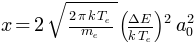# pytexit¶

Convert a Python expression to a LaTeX formula

## Install¶

pytexit is on PyPi:

pip install pytexit


## Use¶

pytexit features the py2tex(), for2tex() and for2py() functions.

In a Terminal, use py2tex:

py2tex 'x = 2*sqrt(2*pi*k*T_e/m_e)*(DeltaE/(k*T_e))**2*a_0**2'


In a Python console, use py2tex():

from pytexit import py2tex
py2tex('x = 2*sqrt(2*pi*k*T_e/m_e)*(DeltaE/(k*T_e))**2*a_0**2')


returns the corresponding LaTeX formula (to re-use in papers):

$$x=2\\sqrt{\\frac{2\\pi k T_e}{m_e}} \\left(\\frac{\\Delta E}{k T_e}\\right)^2 a_0^2$$


and (in ipython console only) prints the equation:## Current Features¶

Successfully deal with most of the one or two parameter functions. Run the _test() function to have an idea of what’s possible.

Arbitrary syntax:

• Variables named after Greek names are turned into LaTeX syntax:

py2tex("Re_x=(rho*v*x)/mu")

$Re_x=\frac{\rho v x}{\mu}$
• ‘numpy.sin / math.sin / np.sin’ syntax still work as expected (all standard scientific module names are removed beforehand):

py2tex('numpy.arccos(x)')

$\begin{split}\\arccos(x)\end{split}$
• quad() is converted into integrals:

py2tex("quad(f,0,np.inf)")

$\int_{0}^{\infty} f(u) du$
• list comprehensions are converted into LaTex syntaX:

py2tex("np.sum([i**2 for i in range(1,101)])==338350")

$\sum_{i=1}^{100} i^2=338350$
• a_subˆsuper variables are converted with “sub” as subscript and “super” as superscript:

py2tex('a_subˆsuper')

$a_{sub}^{super}$

Note that “ˆ” is the circumflex accent instead of the caret sign “^”, and is a valid Python variable name:

a_iˆj=1      # valid in Python3

• complex sub/superscript such as second order sub/superscript and comma are supported:

py2tex('k_i__1_i__2ˆj__1ˆj__2')

\begin{align}\begin{aligned}k_{i_1,i_2}^{j_1,j_2}\\More detailed rules::\\ python -> latex k_i_j -> k_i,j k_i__j -> k_(i_j) k_iˆj -> k_i^j k_iˆˆj -> k_(i^j) k_i__1_i__2ˆj__1ˆˆj__2 -> k_(i_1,i_2)^(j_1,j_2)\end{aligned}\end{align}

Also note that iPython uses auto-completion to convert most of the latex identifiers in their Unicode equivalent:

\alpha --> [press Tab] --> α

• pytexit will recognize those Unicode characters and convert them again in latex expressions:

py2tex('arcsin(α)')

$\arcsin(\alpha)$
• there is a mode to output Python expressions in Word syntax. From version 2007 Word converts most LaTeX expressions in its own graphical representation. The Word mode here was just about replacing those LaTeX {} with Word ():

py2tex('sqrt(5/3)',output='word')


## Test¶

In order to enforce cross-version compatibility and non-regression, pytexit is now tested with pytest and Travis. Run the test suite locally from a terminal with:

pip install pytest
pytest


## Changes¶

• 0.2.1 : full Python 2 support, added automated tests with pytest and Travis
• 0.1.11 : make it reliable: added pytest, Travis, code coverage
• 0.1.8 : fixed console script on Unix systems
• 0.1.4 : partial Python 2 support

## Still WIP¶

Todo:

• allow syntax “a*b = c” (not a valid Python expression, but convenient to type some LaTeX formula)
• code for numbered equations
• export all the conversions on an external text file